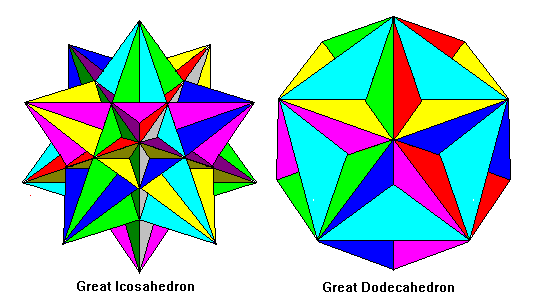# Kepler-poinsot Solids

Steven Dutch, Professor Emeritus, Natural and Applied Sciences, University of Wisconsin - Green Bay

## Star polygons

A regular polygon is one with equal sides and equal vertex angles. Logically, a star is a regular polygon if we allow sides to penetrate through each other. What can we call such a polygon? If we inscribe an n-sided polygon in a circle, its vertices are 360/n degrees apart. To make a five-pointed star, we locate five equally-spaced vertices and connect alternate vertices. The vertices are 360 (2/5) degrees or 144 degrees apart. Thus, we can consider a five-pointed star to be a 5/2-gon. Also, the vertex angles of a regular n-gon are 180(n-2)/n. A regular five-pointed star has vertex angles of 180(5/2 - 2)/(5/2) or 36 degrees.

Exactly this notation is used to describe star polygons. If we create a star by drawing an n-sided polygon and connecting every m vertices, the resulting star is considered an n/m-gon. A 5/3-gon turns out to be the same as a 5/2-gon, 7/3 and 7/4 are the same, and so on.

If m and n have a common factor, like 6/2, a star results (in this case the familiar Star of David) but the edges do not form a continuous set. A Star of David actually consists of two overlapping triangles. Such stars are usually regarded as compounds of polygons rather than true polygons. Cases like 6/3, 8/4, and so on are degenerate "stars" and consist of radiating straight lines

## Stellation

One way to create a star is to draw a polygon, then extend pairs of edges until they meet. This process is called stellation. A natural extension is to extend the faces of a three-dimensional solid (polyhedron) until they meet. The Kepler-poinsot solids are stellations of the dodecahedron and icosahedron.

## The Kepler SolidsOne of the first people in modern times to study polygons, polyhedra, and crystals was the astronomer Johannes Kepler, who discovered the two solids above. In each case, faces of a dodecahedron are extended outward into a star. Both are stellated dodecahedra; they are called "small" and "great" to distinguish them.

The small stellated dodecahedron has twelve five-sided pyramids built on the faces of a dodecahedron. All the triangles are isoceles 36-72-72 degree triangles. Each star face has a pyramid sticking out of its center. The faces are colored to show the stars.

The great stellated dodecahedron also has twelve interpenetrating star faces. It can be thought of as an icosahedron with three-sided pyramids on each face. Again, the triangles are isoceles 36-72-72 triangles. The star faces are harder to see because each star has a rosette of five pyramids in its center. The diagram is colored to show the stars.

## The Poinsot SolidsThe French mathematician Poinsot discovered these elegant shapes. The great dodecahedron has twelve interpenetrating pentagonal faces, each with a five-pointed star in relief. The solid has the edges and vertices of an icosahedron, but instead of triangular faces has triangular dimples. The faces in the dimples are isoceles 36-108-36 degree triangles.

The great icosahedron has twenty equilateral triangle faces that are somewhat hard to see because of their complex intersection. The overall shape is like a small stellated dodecahedron with star-shaped pyramids.

Created 3 Oct. 1997, Last Update 7 June 1999.